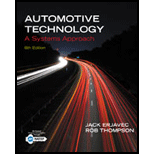Chapter 3, Problem 1RQAutomotive Technology: A Systems A...

6th Edition
Jack Erjavec + 1 other
ISBN: 9781133612315

Solutions

Chapter
SectionAutomotive Technology: A Systems A...

6th Edition
Jack Erjavec + 1 other
ISBN: 9781133612315
Textbook Problem

Describe Newton’s first law of motion and give an application of this law in automotive theory.

To determine

Newton’s first law of motion with an application in terms of automotive theory.

Newton’s first law of motion or Newton’s law of inertia: “Every object in its state of rest or uniform motion(Acceleration is zero) in a straight line unless it is compelled to change that state by forces impressed on it”.

Explanation

When an object is in rest or motion, that object will always be in rest or motion until an external force work on that object.

Example: Assume a car is in rest, the car will be in rest until the force applied. Now the car is moving, and this car will be moving until the force applied on it.

Conclusion:

Newton’s first law of motion is working every condition when any object is in rest or trying to rest.

The Solution to Your Study Problems

Bartleby provides explanations to thousands of textbook problems written by our experts, many with advanced degrees!

Get Started

What does the abbreviation EDM stand for?

Precision Machining Technology (MindTap Course List)

Define each of the following terms: a. data b. field c. record d. file

Database Systems: Design, Implementation, & Management

Give an example of each of the three types of relationships.

Database Systems: Design, Implementation, & Management

Compute the nominal flexural strength of the composite beam in Problem 9.1-3. Use Fy=50 ksi.

Steel Design (Activate Learning with these NEW titles from Engineering!)

Find all the answers to your study problems with bartleby.
Textbook solutions plus Q&A. Get As ASAP arrow_forward